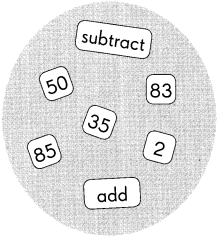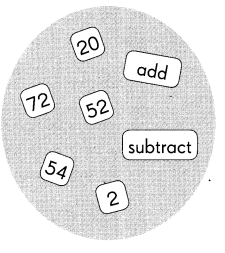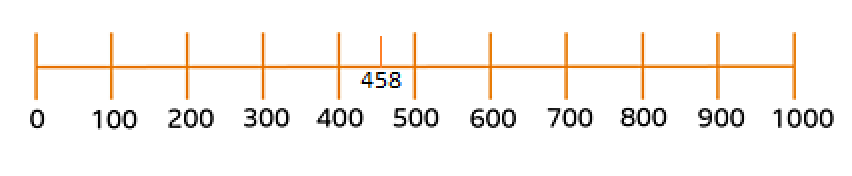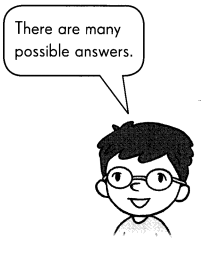# Math in Focus Grade 3 Chapter 2 Answer Key Mental Math and Estimation

Go through the Math in Focus Grade 3 Workbook Answer Key Chapter 2 Mental Math and Estimation to finish your assignments.

## Math in Focus Grade 3 Chapter 2 Answer Key Mental Math and Estimation

Math Journal

Fill in the blanks that show the steps to add or subtract mentally. You may use the numbers more than once.

Question 1.
35 + 48 = ?
Step 1 Add ___ to ___.
____ + ___ = _____
Step 2 Subtract ___ from ___.
____ – ___ = _____
So, 35 + 48 = ____Step 1 Add  35 to 48.
35 + 48 = 83
So, 35 + 48 = 83

Question 2.
72 – 18 = ?
Step 1 Subtract ___ from ___.
____ – ___ = _____
Step 2 Add ___ to ___.
____ + ___ = _____
So, 72 – 18 = ____Step 1 Subtract 72 from  18.
72 – 18 = 54
So, 72 – 18 = 54

Complete.

Question 3.
Explain how to round 458 to the nearest hundred. Include a number line in your explanation.458 is nearest to 5 hundred because the difference between 400 and 458 is greater than 458 and 500.

Question 4.
Use front-end estimation to estimate the difference. Write the steps to your solution and check that your answer is reasonable.
905 – 178
_____________________
_____________________
_____________________
_____________________
_____________________
905 is near to 900
178 is near to 200
So, 900 – 200 = 700
Yes, the above answer is reasonable because 905 – 178 equals 727 which approximately equals 700

Question 5.
John used front-end estimation to estimate the sum of 317 + 268.
Do you agree with his answer? Explain.
317 + 268 = 585
So, 317 + 268 is about 600.
_____________________
_____________________
317 is near to 300
268 is near to 300
So, 300 + 300 = 600.
Yes, I agree with his answer because he followed the correct method and 600 approximately equals 585.

Challenging Practice

Question 1.
Two numbers are rounded to the nearest hundred, then added. The estimated sum is 500. One number is 235. What is the greatest possible value of the other number?
Given,
One number is 235 which is equal to the nearest hundred – 200
So, from given statement – Two numbers are rounded to the nearest hundred, then added. The estimated sum is 500.
Let the other number be X
So, X + 200 = 500
x = 500 – 200 = 300
Therefore, the greatest possible value of the other number is 300.

Question 2.
I am a 3-digit number. When you round me to the nearest ten and to the nearest hundred, the answer is the same. What number can I be?Let the number be 199 and the nearest ten is 200 and the same way the nearest hundred is 200.
So, there are many possible answers – 199, 299, 399, 499, 599, 699, 799, 899, 999

Problem Solving

Mrs. Avilla makes curtains. She needs 356 centimeters of fabric for the kitchen and 517 centimeters for the living room.

Question 1.
Estimate the length of the fabric she needs in all by rounding to the nearest hundred.